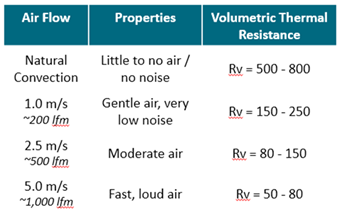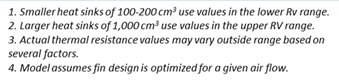﻿ Heat Sink Sizing Calculator V4
 Heat Sink Sizing Calculator - Rough Estimate Estimate the overall volume of the heat sink required to cool a heat source by using the following equation: V= (Q*Rv)/Delta T Heat sink volume in cm3 = (heat source power in watts x volumetric thermal resistance) / (Tjunction - maximum ambient temperature) Inputs (in yellow)Heat Source Power (Q) Watt Tcase Max (manuf supplied) Deg. C Max Ambient Temperature Deg. C Delta - T (Thermal Budget) Deg. C Volumetric Thermal Resistance (Rv) (refer to table at right) Estimated Heat Sink Volume cm3 Desired Heat Sink Volume Length cm Width cm Height (base plus fin) cm Desired Heat Sink Volume cm3 Your Desired Heat Sink Size is Reference https://www.electronics-cooling.com/1995/06/how-to-select-a-heat-sink/